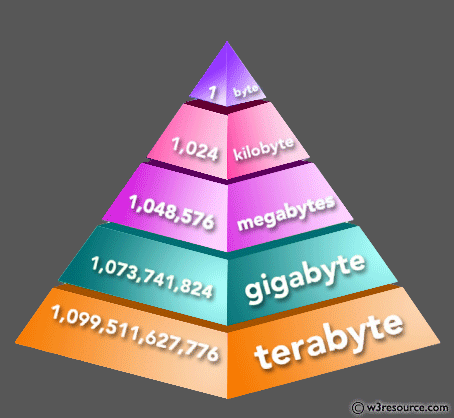﻿ NumPy: Find the memory size of a NumPy array - w3resource# NumPy: Find the memory size of a NumPy array

## NumPy: Array Object Exercise-33 with Solution

Write a NumPy program to find the memory size of a NumPy array.

Pictorial Presentation:Sample Solution:-

Python Code:

``````import numpy as np
n = np.zeros((4,4))
print("%d bytes" % (n.size * n.itemsize))
```
```

Sample Output:

```128 bytes
```

Python Code Editor:

Have another way to solve this solution? Contribute your code (and comments) through Disqus.

What is the difficulty level of this exercise?

Test your Python skills with w3resource's quiz

﻿

## Python: Tips of the Day

Set comprehension:

```>>> m = {x ** 2 for x in range(5)}
>>> m
{0, 1, 4, 9, 16}
```